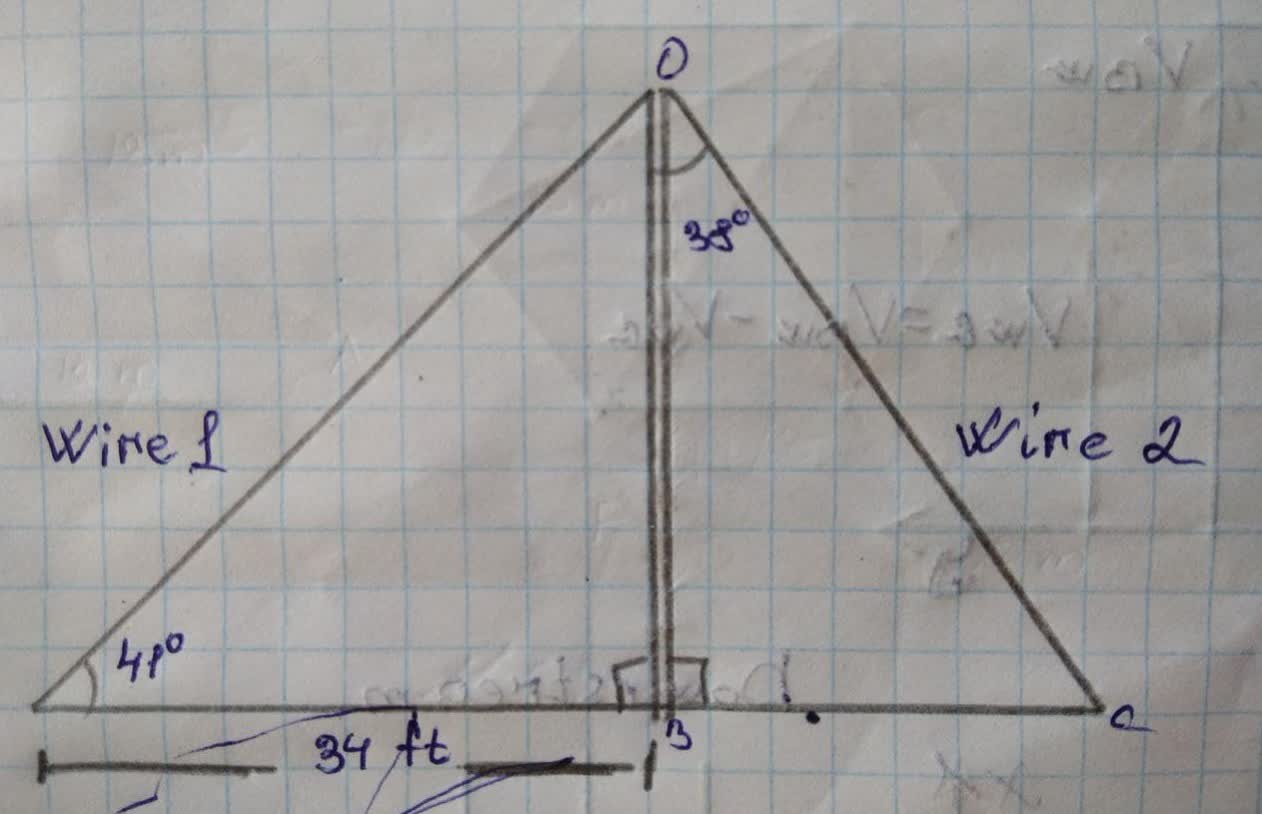A pole has two wires attached to it, one onEdmund Adams 2021-11-28 Answered
A pole has two wires attached to it, one on each side, forming two right triangles as shown.• Questions are typically answered in as fast as 30 minutes

Solve your problem for the price of one coffee

• Math expert for every subject
• Pay only if we can solve itMarlene Broomfield

Step 1
Given that:
A pole has two wires attached to it, one on side forming two right triangles as shown,
From the figure,
Length of pole = OB
$$\displaystyle\angle{O}{A}{B}={41}^{{\circ}}$$
$$\displaystyle\angle{O}{B}{A}={90}^{{\circ}}$$
$$\displaystyle{A}{B}={34}{f}{t}$$
length of wire 1= OA
Step2
Part A
To calculate the length of pole,
In $$\triangle OAB$$,
$$\displaystyle{\frac{{{P}{e}{r}{p}{e}{n}{d}{i}{c}ul{{a}}{r}}}{{{B}{a}{s}{e}}}}={\tan{{\left({41}^{{\circ}}\right)}}}$$
$$\displaystyle{\frac{{{O}{B}}}{{{A}{B}}}}={\tan{{\left({41}^{{\circ}}\right)}}}$$
$$\displaystyle{O}{B}={A}{B}\times{\tan{{\left({41}^{{\circ}}\right)}}}$$
$$\displaystyle={34}\times{\tan{{\left({41}^{{\circ}}\right)}}}$$
$$\displaystyle={29.56}{f}{t}$$
Hence the poll is 29.56 ft tall
Step3
Part B
To calculate the length of wire 1,
Apply Pythagoras theorem in triangle OAB,
In $$\triangle OAB$$,
$$\displaystyle{\left({O}{A}\right)}^{{{2}}}={\left({O}{B}\right)}^{{{2}}}+{\left({A}{B}\right)}^{{{2}}}$$
$$\displaystyle={\left({29.56}\right)}^{{{2}}}+{\left({34}\right)}^{{{2}}}$$
$$\displaystyle={873.7936}+{1156}$$
$$\displaystyle{\left({O}{A}\right)}^{{{2}}}={2029.7936}$$
$$\displaystyle{O}{A}=\sqrt{{{2029.7936}}}$$
$$\displaystyle{O}{A}={45.05}{f}{t}$$
Hence wire 1 is 45.05 ft long